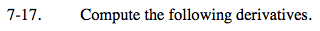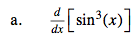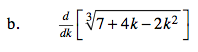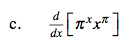### Home > CALC > Chapter 7 > Lesson 7.1.2 > Problem7-17

7-17.sin3(x) = (sin(x))3$\sqrt{7+4k-2k^{2}}=(7+4k-2k^{2})^{1/3}$

$\frac{1}{3}(7+4k-2k^{2})^{-2/3}(4-4k)$